You are using an outdated browser. Please upgrade your browser to improve your experience.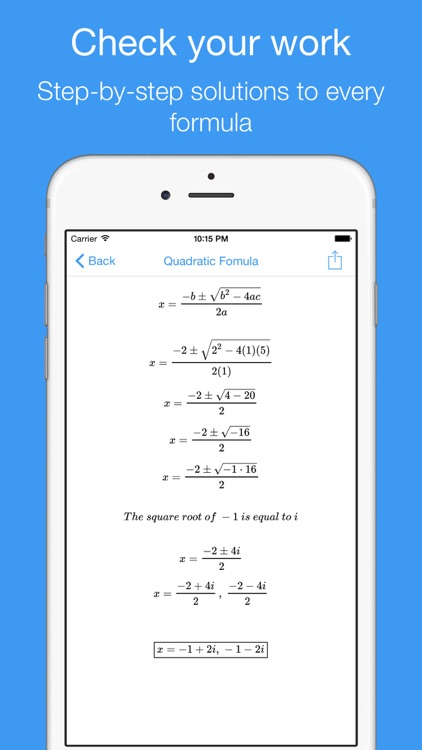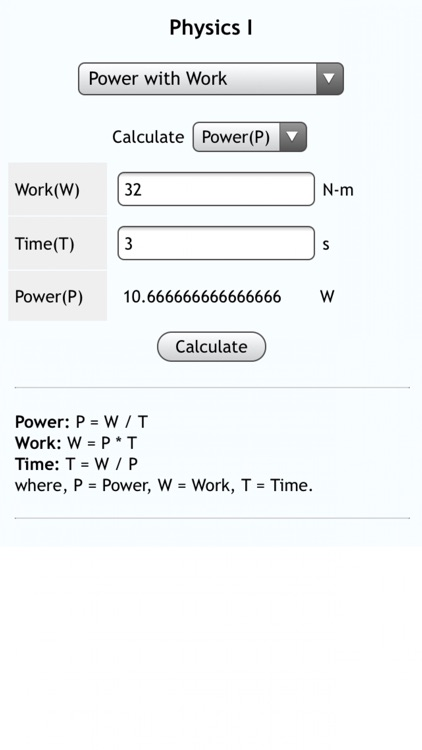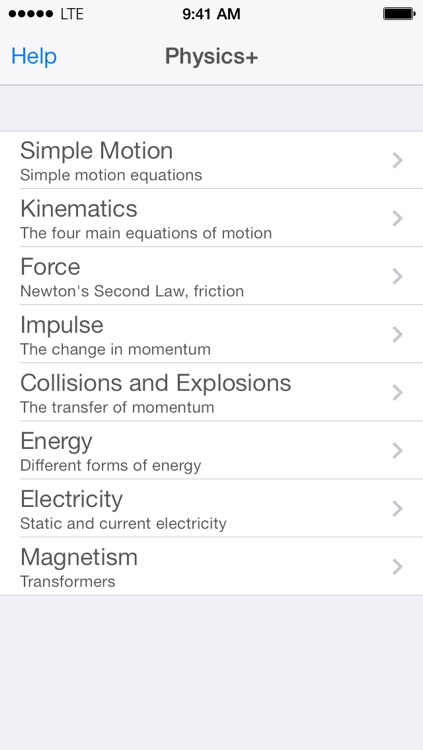Best Apps

Highest scored apps in the category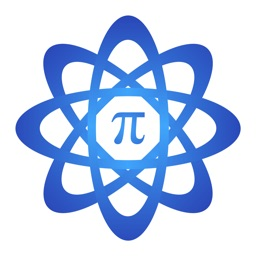MathKit

Black & Grey Studios

MathKit is your all-in-one math and physics formula solver

MathKit is your all-in-one math and physics formula solver! Like your very own personal tutor, MathKit walks you through formulas step-by-step and displays the work as you would write it. Other advanced calculators are archaic and difficult to navigate, but with MathKit’s intuitive user interface, the answers are only seconds away.

• Solves for any variable in over 100 math and...

Also Good

Apps with average score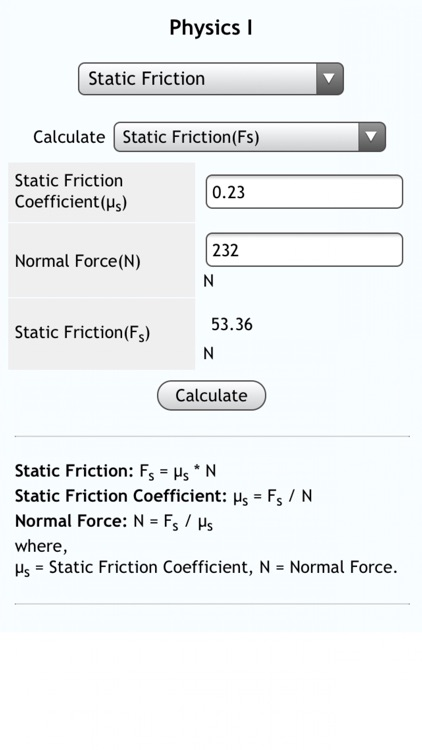Physics I

Darren Gates

Whether you're taking a class in physics, performing research, or just enjoy science, this multifunctional calculator is indispensable. In the palm of your hand, you instantly have access to a ton of specific equations: just plug in the variables and press the Calculate button.

Includes equations for acceleration, displacement, force,...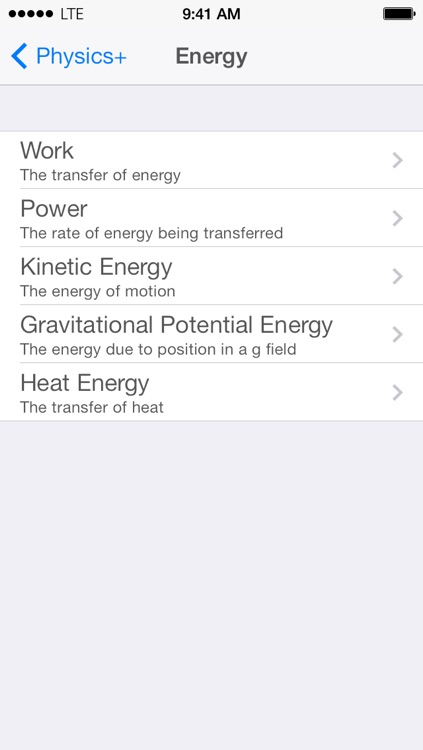Physics+

Jack Stone

The successor to Kinematics+, Physics+ is here. Physics+ is one of the few calculators that you can talk to and learn from. That's right, you can talk to it.

Physics+ presents a well-designed, elegant interface designed by a high school physics student that is specifically tailored to high school physics students....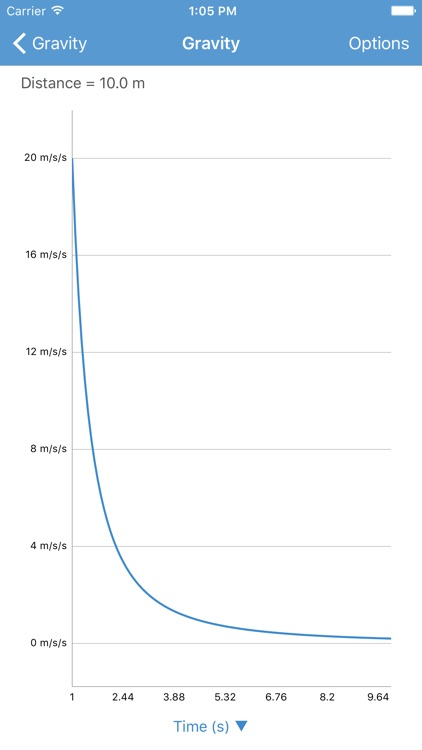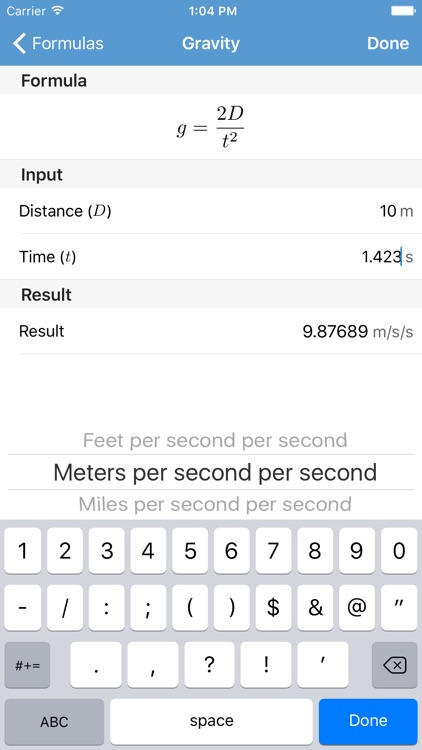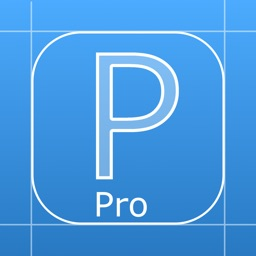Physics! Pro

Erway Software

Physics! Pro is a simple, intuitive physics calculator which can find:

- Force, Mass, and Acceleration

- Momentum, Mass and Velocity of a moving object

- Period and Frequency of a Pendulum

- Distance, Time and Gravity

- Potential and Kinetic Energy

- The force of gravity between two objects

...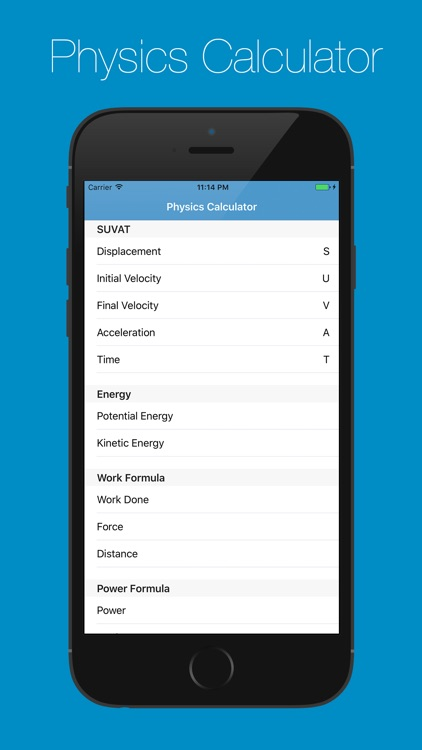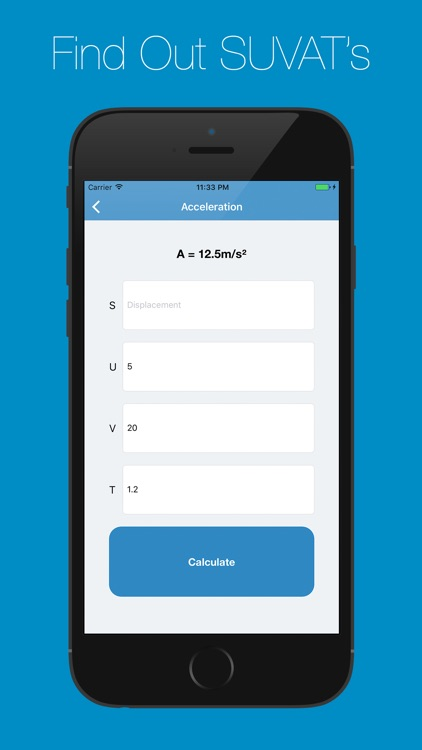Physics Calculator

Henry Goodwin

Physics Calculator is a simple way to quickly and easily find answers to you physics equation. Use Physics Calculator to find displacement (s), initial velocity (u), final velocity (v), acceleration (a) and time (t) using the SUVAT method or use the app to find out Kinetic or Potential Energy along...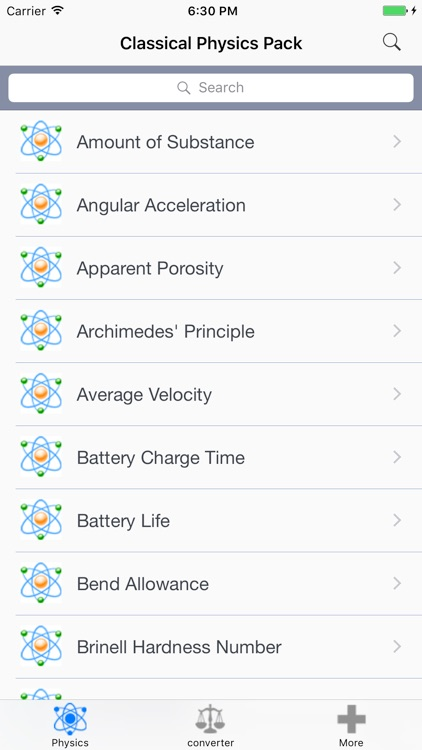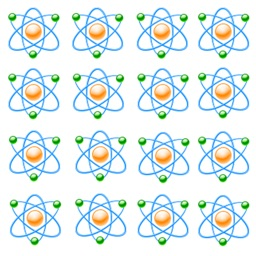Classical Physics Pack

V PUGAZHENTHI

Classical Physics Pack consists of 134 Physics Calculators & 54 Physics Converters.

** Available in English, Français, Español, Italiano, Deutsch & Português **
Physics Calculator:
• Force
• Kinetic Friction
• Static Friction
• Centripetal Force
• Centripetal Acceleration
• Gravitational Acceleration
• Angular Acceleration
• Work
• Total Work
• Power with...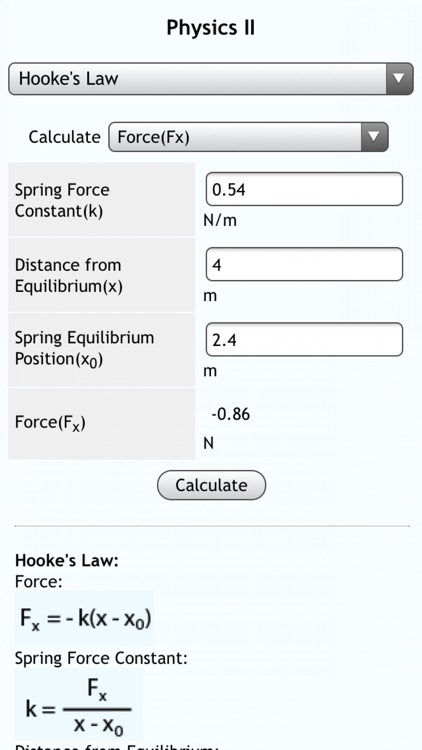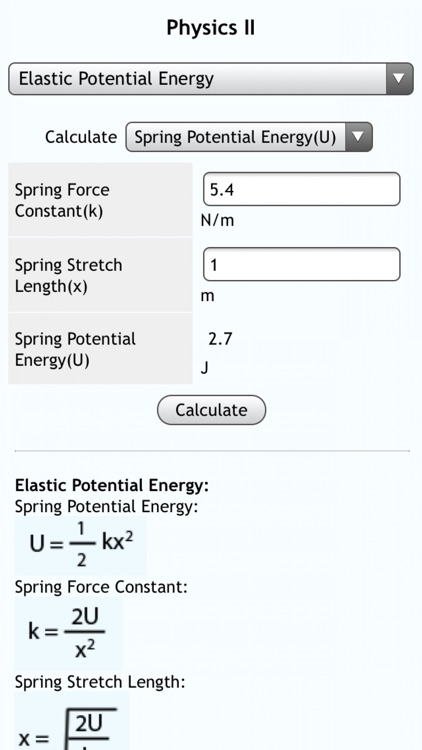Physics II

Darren Gates

Whether you're taking a class in physics, performing research, or just enjoy science, this multifunctional calculator is indispensable. In the palm of your hand, you instantly have access to a ton of specific equations: just plug in the variables and press the Calculate button.

Includes equations for density, mass energy,...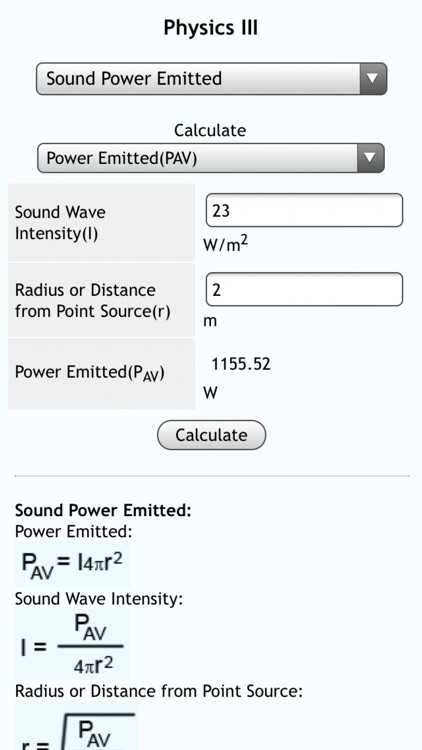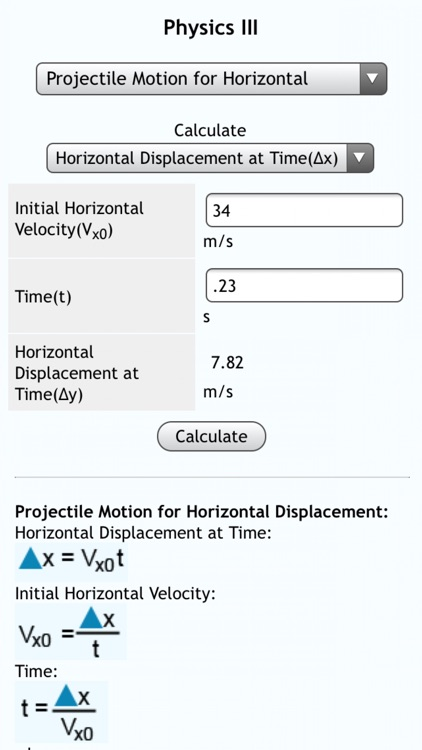Physics III

Darren Gates

Whether you're taking a class in physics, performing research, or just enjoy science, this multifunctional calculator is indispensable. In the palm of your hand, you instantly have access to a ton of specific equations: just plug in the variables and press the Calculate button.

Includes equations for battery life, Celsius to...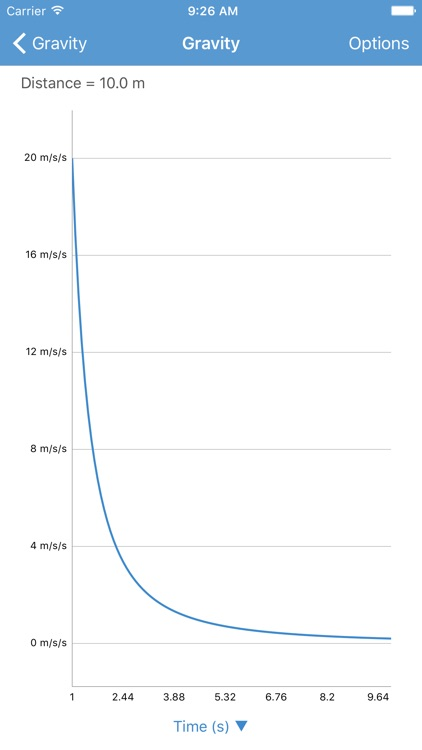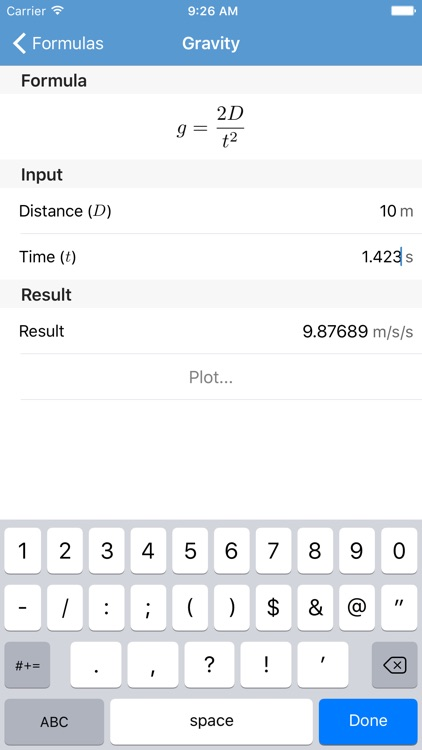Physics!

Erway Software

Physics! is a physics calculator which allows you to find:

- Force, Mass and Acceleration

- Momentum, Mass and Velocity of a moving object

- Period and Frequency of a Pendulum

- Distance, Time and Gravity

- Pressure, Area and Force

- Potential Energy

- Kinetic Energy

- Velocity, Wavelength and Frequency for Waves

The app uses SI...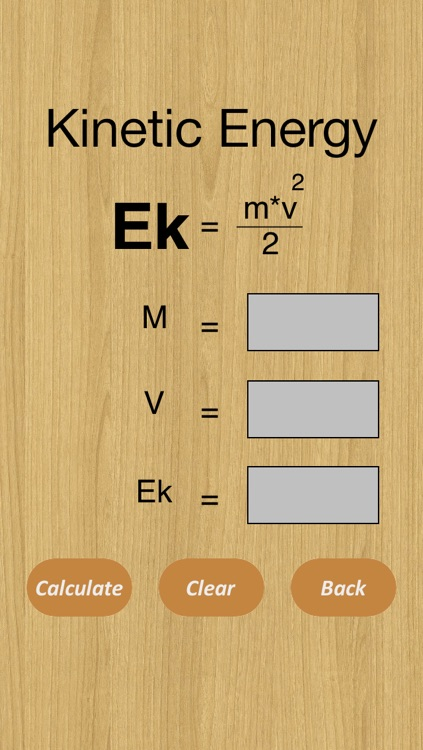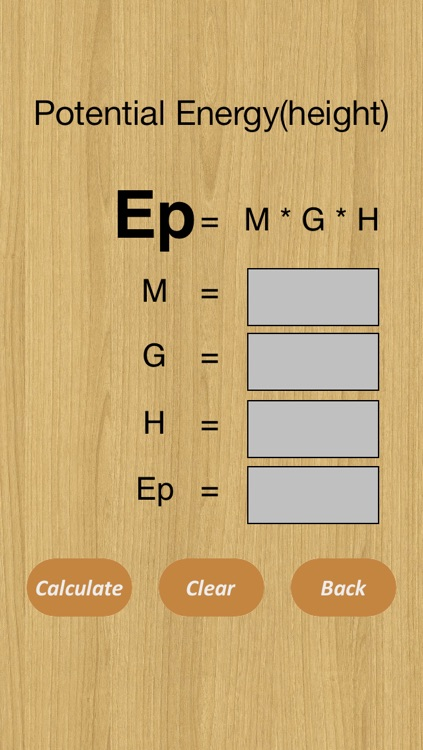Physics Formulas Calculator

iThink Different TLDC

This Application will help you to solve physics formulas in the most useful and the fastest way!
The Application contain 2 formulas:
1.Kinetic Energy Formula
2.Potential Energy Formula
We will provide more Formulas in the future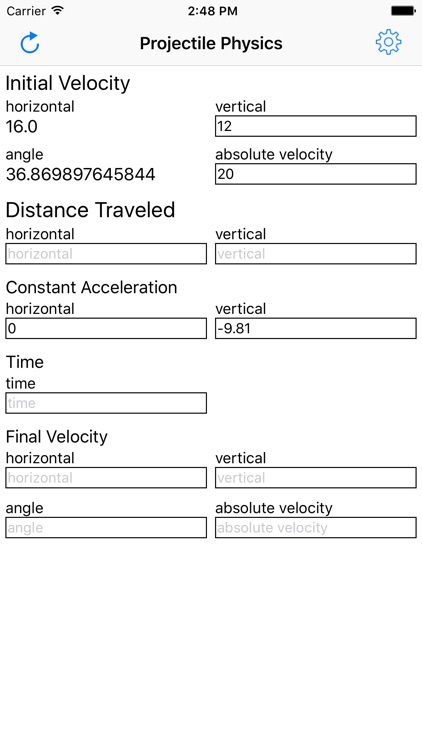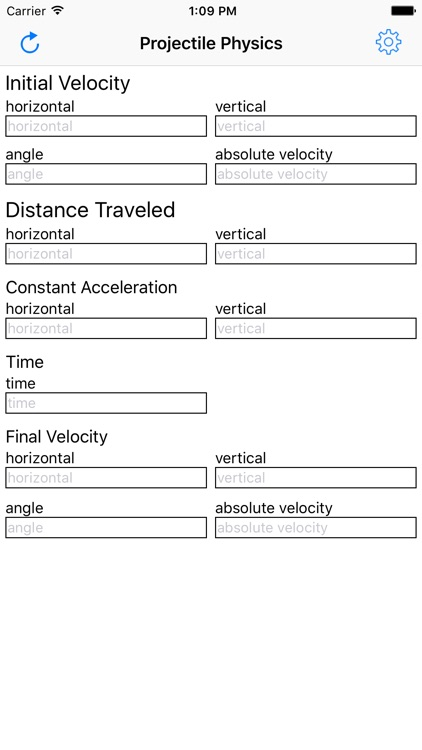Projectile Physics

Ebscer

A calculator that helps solve projectile motion physics problems.

This program solves for distance, acceleration, time, and initial and final velocity. All equations are solved in real time, allowing you to quickly switch between focusing on different aspects of the model.